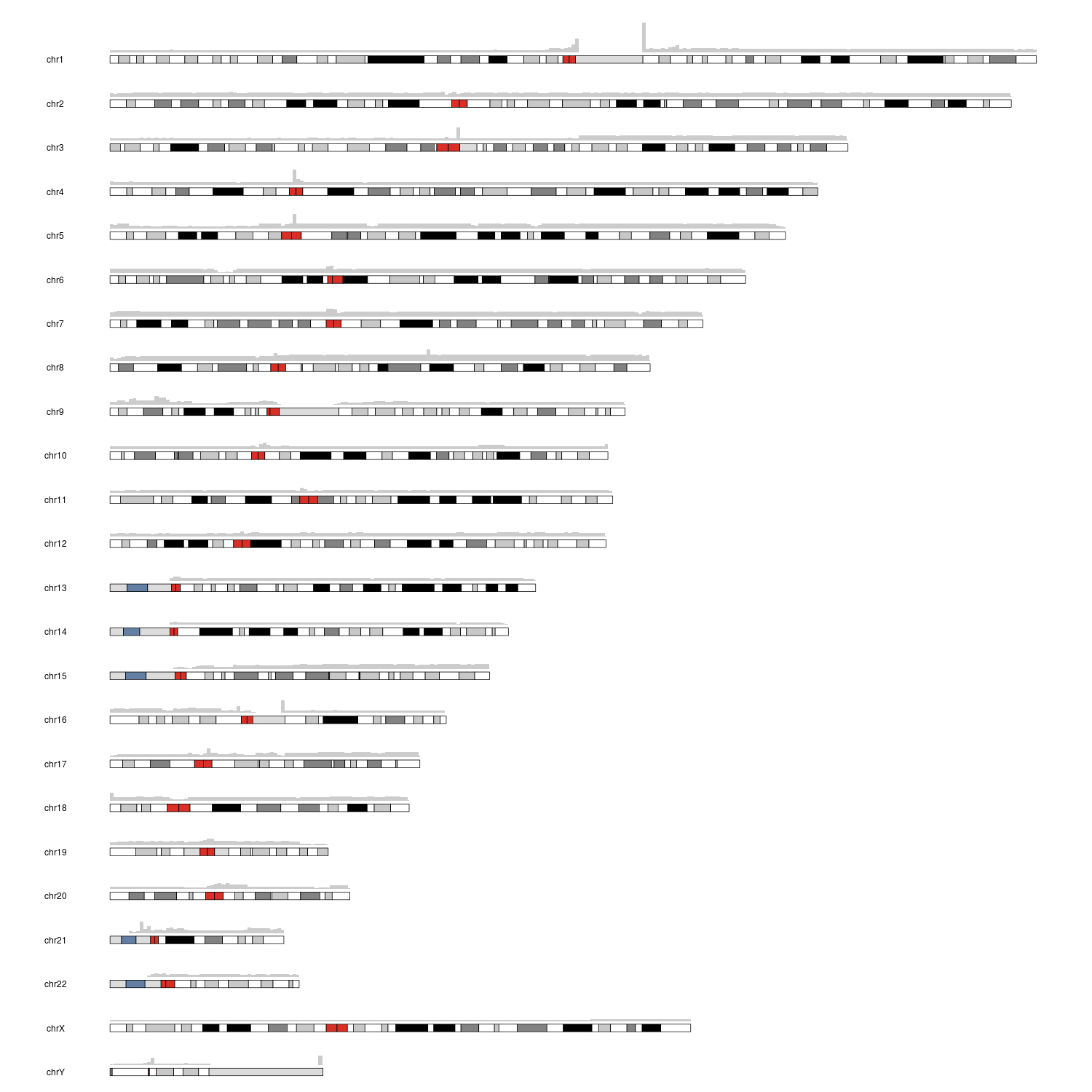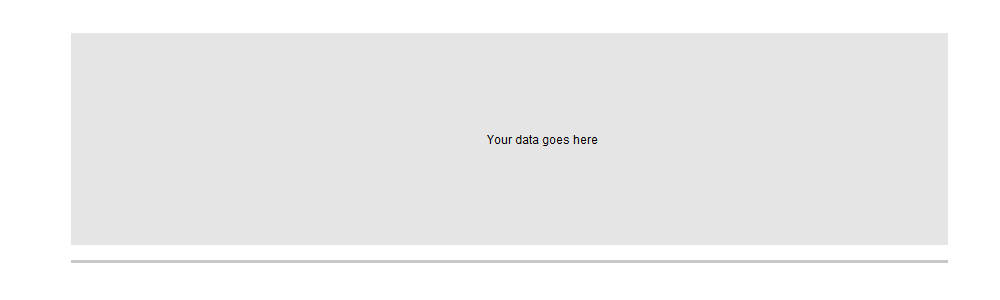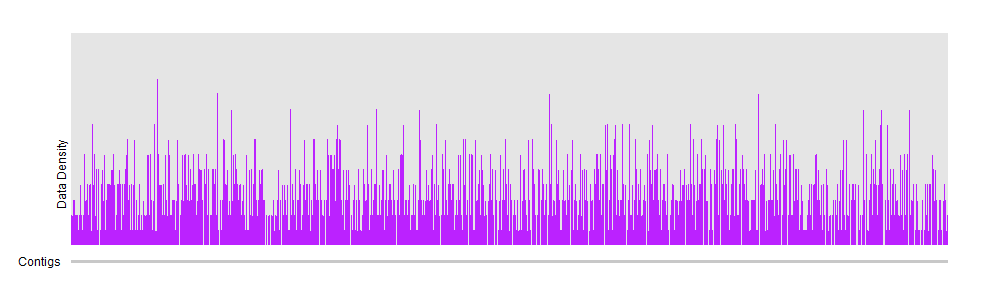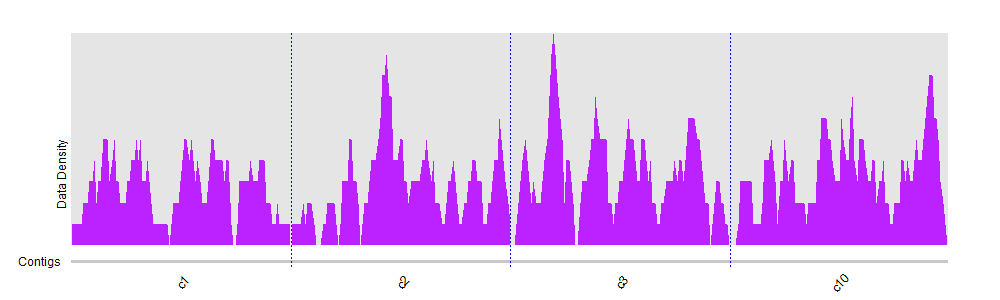genome coverage visualization
1
1
Entering edit mode
2.8 years ago
gubrins ▴ 270

Heys,

I'm working with whole-genome sequencing data and I already did the alignment and I have my bam files. I calculated the coverage per genome position of those bam files with bedtools:

bedtools genomecov -ibam file.bam -d > coverage.txt


My problem is that coverage.txt is 50GB. I tried to visualize this with R in the cluster but it runs out of memory. So my doubts: do you know how could I reduce the size of this file? Maybe there is a better way to calculate and visualize genome coverage?

Any help would be appreciated!

bam genome coverage bedtools • 6.6k views
1
Entering edit mode

You can try to divide and compute mean coverage for regions instead of trying to visualize individual positions. You can find some other ideas here.

0
Entering edit mode

Thanks for your answer, the problem is that I don't have identified regions yet, so it would be problematic

4
Entering edit mode
2.8 years ago
bernatgel ★ 3.4k

Hi Gabri,

You should be able to do that with karyoploteR.

If you want a full genome view of the coverage, I'd recommend you to plot a binned version of the coverage instead of a per base value since you won't have enough pixels in your image for that kind of resolution anyway. With karyoploteR I'd use the kpPlotBAMDensity function, that will take a BAM file directly. compute the read density with the window sizes you specify (defaults to 1 megabase windows), so 3000 data points for a human genome, and plot them on the karyotype. For example, to plot the coverage in a full genome with default 1Mb windows you can do something like

library(karyoploteR)

kp <- plotKaryotype(genome="hg38")
kpPlotBAMDensity(kp, data="yourBAMfile.bam")


and that's it. It will take a while (that'a lot of data to process) but it will do it sequentially and never load the whole dataset into memory, so eventually it will end and generate an image like this:You can also restrict the plot to a single chromosome or zoom in to a small region in the genome. or add other data onto it. You can find more information in the karyoploteR tutorial although kpPlotBAMDensity is still missing from it.

0
Entering edit mode

Thank you very much Bernat for your complete answer, your package is awesome! The problem for me is that I'm not working with human data, and my reference genome has a huge amount of contigs, so visualizing like this would be problematic. For this reason I was trying to get a simple plot with coverage on X and number of bases on Y. I already did that for individual contigs, but I have too many. Any ideas of how could I plot it? I tried in a computer with 100GB of RAM but didn't made it... Thanks!

2
Entering edit mode

Hi Gabri, karyoploteR can work with any genome, or even a collection of contigs, if needed. I agree that this representation does not work for a large number of chromosomes/contigs, but it can plot them one next to the other in a horizontal line. To do that, you'll need to set the plot.type=4 in plotKaryotype.

Since computing the density from BAM files is sequential, it should not take a huge amount of RAM.

As an example, I created a fake genome with 1000 contings oz sizes between 10Kb and 100Kb.

library(karyoploteR)
cont.length <- sort(runif(n = 1000, min = 10000, max=100000), decreasing = TRUE)
gg <- toGRanges(data.frame(paste0("c", 1:1000), rep(1, 1000), cont.length))


and we can plot them with plot.type=4. We should adjust a few plotting parameters to get what we want.

pp <- getDefaultPlotParams(plot.type = 4)
pp$ideogramlateralmargin <- 0 pp$leftmargin <- 0.07

kp <- plotKaryotype(genome = gg,  plot.type=4, ideogram.plotter = NULL, labels.plotter = NULL, plot.params = pp)
kpDataBackground(kp)
kpText(kp, chr="c350", x=1, y=0.5, labels = "Your data goes here")and we can plot the density on top of it (in this case the density of a set of random regions)

rr <- createRandomRegions(nregions = 30000, length.mean = 5000, length.sd = 2000, genome=gg, non.overlapping = FALSE)

kp <- plotKaryotype(genome = gg,  plot.type=4, ideogram.plotter = NULL, labels.plotter = NULL, plot.params = pp)
kpDataBackground(kp)
kpPlotDensity(kp, data=rr, col="#BB22FF", window.size = 1000, border=NA)and you can then show a handful of contigs if needed for better analysis

kp <- plotKaryotype(genome = gg, chromosomes=c("c1", "c2", "c3", "c10"), plot.type=4, ideogram.plotter = NULL, labels.plotter = NULL, plot.params = pp)
kpDataBackground(kp)
kpPlotDensity(kp, data=rr, col="#BB22FF", window.size = 1000, border=NA)1
Entering edit mode

Thanks again for your great answer! Although is not exactly what I need right now, I'm sure I'll use it in the future for checking the coverage of specific regions of my interest! Really interesting package, thanks again!

0
Entering edit mode

Hi @bernatgel, KaryoploteR is a must-try tool. Thanks! I am trying to plot chr9, chr1, and chr2 to see the average coverage. Is there a way to do so using data= a bam file?

0
Entering edit mode

Hi shruti.s

I don't know if I correctly get your question. If you want to plot only a handful of chromosomes, you need to add "chromosomes=c("chr9", "chr1", "chr2")" to plotKaryotype. You can see more information about this in the karyoploteR tutorial.

If you want to plot BAM coverage along whole chromosomes (as opposed to small regions) I'd recommend using kpPlotBAMDensity instead of kpPlotBAMCoverage since it'll be much faster and use less memory.

If you want karyoploteR to compute the mean coverage of each chromosome, I'm afraid karyoploteR cannot do this, since it is a plotting library and nothing else.You should use another software for that kind of computations.

Hope this helps